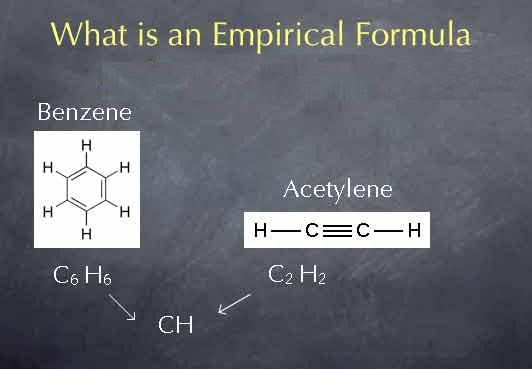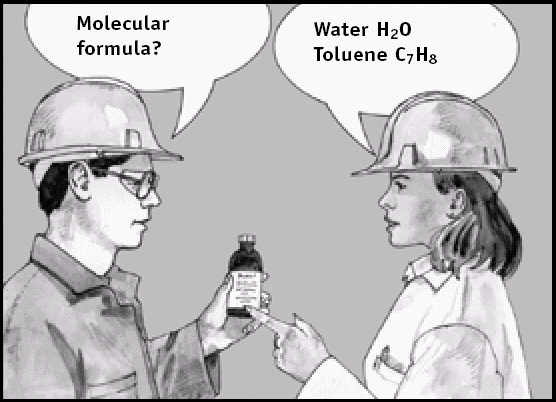# Difference Between Empirical and Molecular Formula

In the world of chemistry, compounds can be expressed in different ways. You can use their chemical formulas or can also call them using their common names. However, chemical formulas are commonly used to express compounds, as the chemical formulas contain much more information about the atoms that combine to make up a compound.

The chemical formulas are then divided into two types, which are empirical formulas and the molecular formulas. Both the formulas clearly show which elements combine together to make up a compound. However, the difference between the molecular formula and the empirical formula is  in their subscripts.

The usage of the type of chemical formula depends on the requirements. In high school text books, the students are taught about both the chemical formula types, so that they can understand the real difference between the both.

In molecular formula, the subscripts describe the actual number of atoms present in a particular molecule. On the other hand, the subscripts in the empirical formula explain the whole number ratio between the atoms that form a particular chemical compound.

For instance, Hexane is a chemical compound that requires six atoms of carbon and 14 atoms of hydrogen to make a single molecule. So, the molecular formula for Hexane will be C6H14, whereas, the empirical formula will be C3H7.

Most of the high school text books use empirical formula, as they also explain the ionic compounds and macromolecules. When different molecules are held together due to electrostatic force, they form ionic compounds. In the meantime, the macromolecules are large molecules. Therefore, if they are not expressed using empirical formulas, then there is a chance that the subscript may become huge and it will become really difficult to write them.

Empirical formulas can also be applied in Physics. So, empirical formulas have versatile usage compared to the molecular formulas. In physics, empirical formulas are used to form mathematical equations. When a physicist tests on atom’s movements and their interaction with each other, they can use such equations to predict the observable results.

### Instructions

• 1

Empirical formula

Empirical formula is used to express chemical compounds using the whole number ratio of the atoms present in a compound or a substance.

Image courtesy: chemcollective.org• 2

Molecular formula

Molecular formula is used to express chemical compounds, using the exact ratio of the atoms present in a compound or a substance.

Image courtesy: reptox.csst.qc.ca•